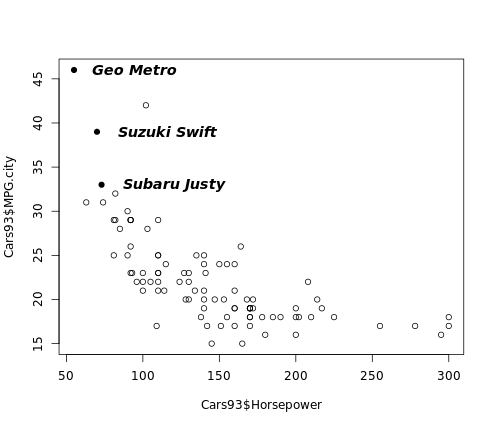# PCH in R Tutorial

In this tutorial, learn about plot character (PCH) in R.

Plot character or pch is the standard argument to set the character that will be plotted in a number of R functions.

Explanatory text can be added to a plot in several different forms, including axis labels, titles, legends, or a text added to the plot itself. Base graphics functions in R typically create axis labels by default, although these can be overridden through the argument `xlab()` that allows us to provide our own x-axis label and `ylab()` that allows us to provide our own y-axis label.

Some base graphics functions also provide default titles, but again, these can be overridden has we see here:

``````library(MASS)
par(mfrow = c(1, 2))
plot(density(geyser\$waiting))
plot(density(geyser\$waiting), main = "Estimated density: \n Old Faithful waiting times")
``````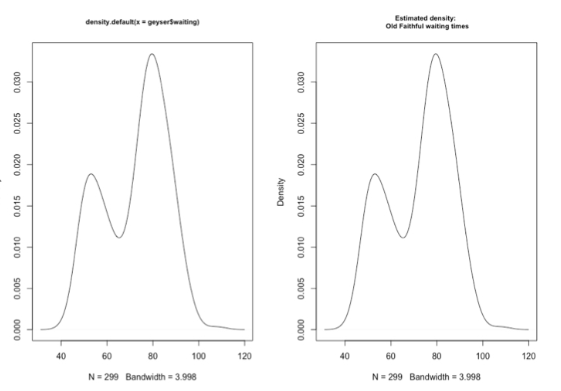The plot on the left uses the default title returned by the density function, which tells us that the plot was generated by this function using its default options and also gives the R specification for the variable whose density we are plotting. In the right-hand plot, this default title has been overridden by specifying the optional argument main. Note that by including the return character, backslash n, we are creating a two-line title in this character string.

## `text()` Function

Like the lines and points functions, text is a low-level graphics function that allows us to add explanatory text to the existing plot. To do this, we must specify the values for x and y, the coordinates on the plot where the text should appear, and labels, a character vector that specifies the text to be added.

``````text(x, y, adj)
``````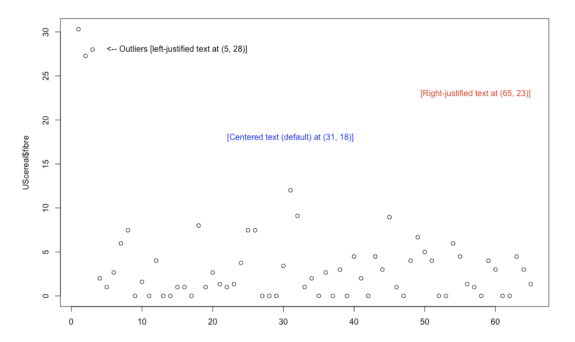By default, the text added to the plot is centered at the specified x values, but the optional argument `adj` can be used to modify the alignment.

It is noted that the `adj` argument to the `text()` function determines the horizontal placement of the text, and it can take any value between 0 (left-justified text) and 1 (right-justified text). In fact, this argument can take values outside this range. That is, making this value negative causes the text to start to the right of the specified `x` position. Similarly, making `adj` greater than 1 causes the text to end to the left of the `x` position.

``````library(MASS)
plot(UScereal\$fibre)
text(5, 28, "<-- Outliers [left-justified text at (5, 28)]", adj = 0)
text(65, 23, "[Right-justified text at (65, 23)]", adj = 1, col = "red")
text(5, 28, "[Centered text (default) at (31, 18)]", col = "blue")
``````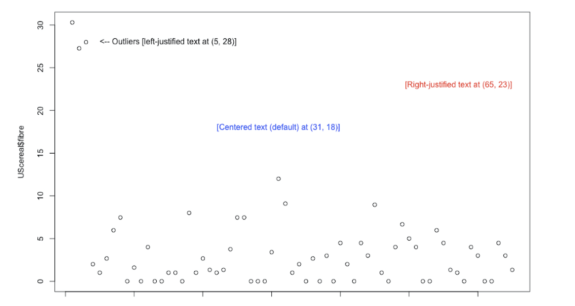### Fonts, Orientations, and Other Text Features

Below we see some of the ways the appearance of added text can be changed through optional arguments to the text function. By default, text is created horizontally across the page, but this can be changed with the `srt` argument, which specifies the angle of orientation with respect to the horizontal axis.

Next, the text in red angles upward as a result of specifying `srt` as the positive value 30 degrees, while the text in green angles downward by specifying `srt` equals -45.

We can also specify the color of the text with the `col` argument, and the red text shows that we can change the text size with the `cex` argument. By specifying `cex = 1.2`, we have specified the text to be 20% larger than normal.

The other text characteristic that has been modified in this example is the font, set by the `font` argument. The default value is `font = 1`, which specifies normal text, while `font = 2` specifies boldface, `font = 3` specifies italics, and `font = 4` specifies both boldface and italics.

``````library(MASS)

# "Inner city" with adjusted colour and rotation
text(350, 24, adj = 1, "Inner city? -->", srt = 30, font = 2, cex = 1.2, col = "red")

# "Suburbs" with adjusted colour and rotation
text(100, 15, "Suburbs? -->", srt = -45, font = 3, col = "green")

title("Text with varying orientations, fonts, sizes & colors")
``````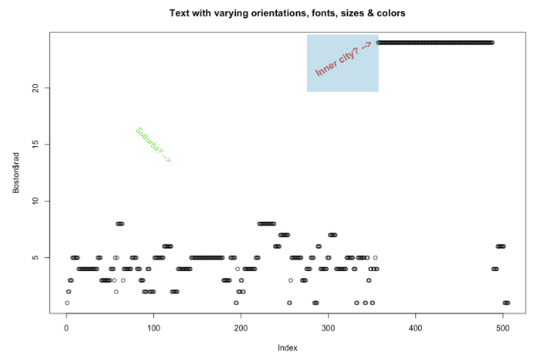## Adjusting Text Position, Size, and Font

In the following example, you will:

• Create a plot of `MPG.city` vs. `Horsepower` from the `Cars93` data frame, with data represented as open circles (default `pch`).
• Construct the variable `index3` using the `which()` function that identifies the row numbers containing all 3-cylinder cars.
• Use the `points()` function to overlay solid circles, `pch = 16`, on top of all points in the plot that represent 3-cylinder cars.
• Use the `text()` function with the `Make` variable as before to add labels to the right of the 3-cylinder cars, but now use `adj = 0.2` to move the labels further to the right, use the `cex` argument to increase the label size by 20 percent, and use the `font` argument to make the labels bold italic.
``````# Plot MPG.city vs. Horsepower as open circles
plot(Cars93\$Horsepower, Cars93\$MPG.city)

# Create index3, pointing to 3-cylinder cars
index3 <- which(Cars93\$Cylinders == 3)

# Highlight 3-cylinder cars as solid circles
points(Cars93\$Horsepower[index3],
Cars93\$MPG.city[index3],
pch = 16)

# Add car names, offset from points, with larger bold text
text(Cars93\$Horsepower[index3],
Cars93\$MPG.city[index3],
Cars93\$Make[index3],
adj = -0.2, cex = 1.2, font = 4)
``````

When we run the above code, it produces the following result: